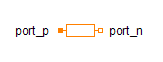Sum - MapleSim Help

Integer Sum

Sum of IntegersDescription The Integer Sum (or Sum) component computes the scaled sum of the elements of the input integer vector.Equations $y={k}_{1}{u}_{1}+{k}_{2}{u}_{2}+\cdots +{k}_{{n}_{u}}{u}_{{n}_{u}}$Connections

 Name Description Modelica ID $u$ Vector of Integer input signals u $y$ Integer output signal yParameters

 Name Default Units Description Modelica ID $k$ $\mathrm{fill}\left(1,{n}_{u}\right)$ Input gains k ${n}_{u}$ $2$ Size of input vector nuModelica Standard Library The component described in this topic is from the Modelica Standard Library. To view the original documentation, which includes author and copyright information, click here.